Function Repository Resource:

Generate the comrade matrix corresponding to an orthogonal polynomial series

Contributed by: Jan Mangaldan
 ResourceFunction["ComradeMatrix"][cof,poly] yields the comrade matrix corresponding to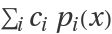, where pi(x) is an orthogonal polynomial represented by poly and ci is the (i+1)th element of the list cof.

## Details

A comrade matrix is an upper Hessenberg matrix whose characteristic polynomial is a scalar multiple of the sum.
The argument poly can be any of the following:
 "ChebyshevFirst" Chebyshev polynomial of the first kind ChebyshevT[i,x] "ChebyshevSecond" Chebyshev polynomial of the second kind ChebyshevU[i,x] "Hermite" Hermite polynomial HermiteH[i,x] "Laguerre" Laguerre polynomial LaguerreL[i,x] "Legendre" Legendre polynomial LegendreP[i,x] {"Gegenbauer",m} Gegenbauer polynomial GegenbauerC[i,m,z] {"Laguerre",a} Jacobi polynomial JacobiP[i,a,b,x] {"Jacobi",a,b} Jacobi polynomial JacobiP[i,a,b,x]
Strings in specifications like "Legendre" and {"Jacobi",a,b} can be replaced with the corresponding built-in symbols, as in LegendreP and {JacobiP,a,b}.
If poly is "ChebyshevFirst", the comrade matrix is also referred to as a colleague matrix.
The result of ResourceFunction["ComradeMatrix"] is a SparseArray.

## Examples

### Basic Examples (3)

The comrade matrix of a Legendre series:

 In:=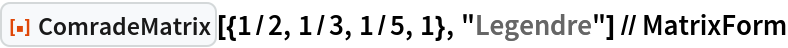Out=Compute its characteristic polynomial:

 In:=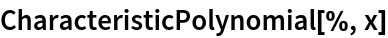Out=The characteristic polynomial is a scalar multiple of the corresponding orthogonal polynomial series:

 In:=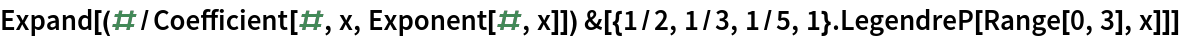Out=### Scope (2)

Comrade matrix of a Jacobi series with symbolic coefficients and parameters:

 In:=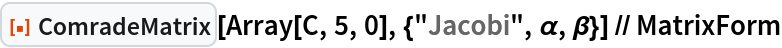Out=An equivalent specification:

 In:=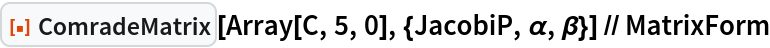Out=Generate a colleague matrix:

 In:=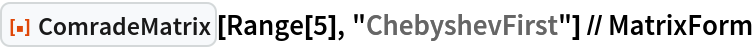Out=### Applications (2)

Use the comrade matrix with Eigenvalues to find the roots of a Chebyshev series of the second kind:

 In:=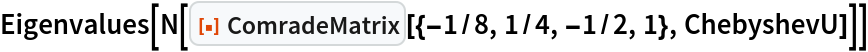Out=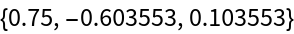Compare with the result of using NSolve and the resource function OrthogonalPolynomialSum:

 In:=Out=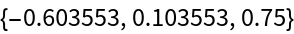## Version History

• 1.0.0 – 05 April 2021